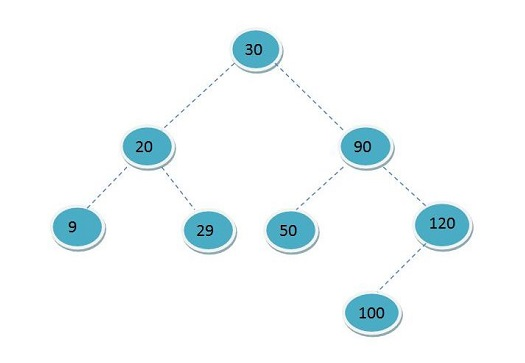# C++ print Postorder traversal from Preorder and Inorder traversal of a tree

In this tutorial, we will learn how to print postorder traversal from preorder and inorder traversal of a tree using C++ program? By Shubham Singh Rajawat Last updated : August 06, 2023

## Problem statement

Given Preorder and Inorder traversal of a tree, write a C++ program to print Postorder traversal.

Consider the below-given example with sample input and output -```Inorder traversal of the given tree is:
- 9, 20, 29, 30, 50, 90, 100, 120

Preorder traversal of the given tree is:
- 30, 20, 9, 29, 90, 50, 120, 100

Postorder traversal of the given tree is:
- 9, 29, 20, 50, 100, 120, 90, 30
```

## Algorithm

As we know that in preorder traversal root is always the first element so we will find the left and right subtree recursively and at last the root, for that we have to search inorder for the left and right subtree. First we will search elements of preorder in inorder as the elements after the index of the searched element in inorder are the members of the right subtree and elements before the index are elements of right subtree and the searched element is the root of that subtree.

## C++ program to print Postorder traversal from Preorder and Inorder traversal of a tree

```#include <iostream>
using namespace std;

// Find x in inOrder
int search(int arr[], int x, int n)
{
int i;
for (i = 0; i < n; i++) {
if (arr[i] == x)
return i; // return the index of the element found
}
return -1;
}

void printPostOrder(int inOrder[], int preOrder[], int n)
{
int rootNode = search(inOrder, preOrder, n);
if (rootNode) // If right subtree is not empty
{
printPostOrder(inOrder, preOrder + 1, rootNode);
}
if (rootNode != n - 1) // If left subtree is not empty
{
printPostOrder(inOrder + rootNode + 1, preOrder + rootNode + 1, n - rootNode - 1);
}
cout << preOrder << " ";
}

// Main code
int main()
{
int inOrder[] = { 9, 20, 29, 30, 50, 90, 100, 120 };
int preOrder[] = { 30, 20, 9, 29, 90, 50, 120, 100 };

int n = sizeof(inOrder) / sizeof(inOrder);

cout << "Post order : ";
printPostOrder(inOrder, preOrder, n);
cout << endl;

return 0;
}
```

## Output

```Post order : 9 29 20 50 100 120 90 30
```